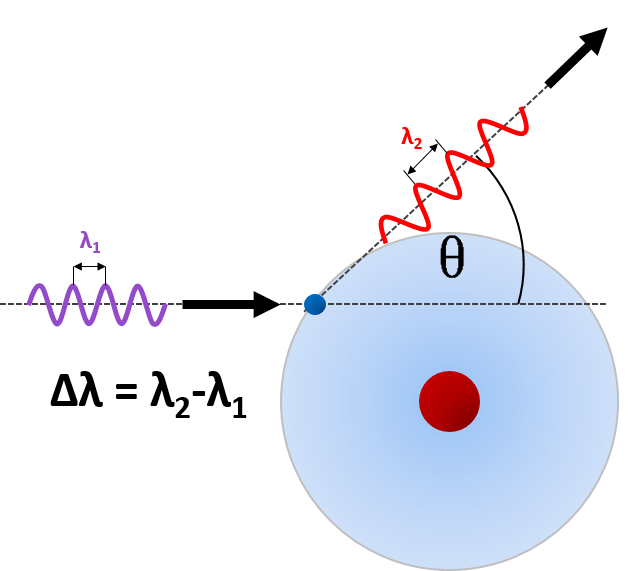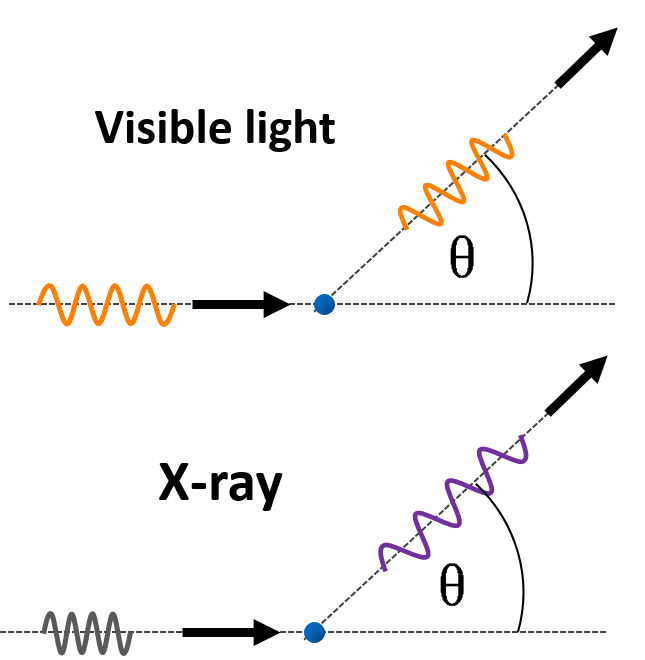# Compton Scattering Calculator

Created by Wojciech Sas, PhD candidate
Reviewed by Bogna Szyk
Last updated: May 18, 2020

Compton scattering calculator is a tool which helps you to find the wavelength extension of a photon scattered by any angle in this particular case. Compton effect is among the possible interactions between light and matter. After this process the wavelength of the photon increases and the traveling direction changes. It is one of the phenomena which confirm the corpuscular nature of light.

## Scattering process

A single photon can be treated as a massless particle which transports energy depending on its wavelength or frequency. When the photon collides with another particle, let's say an electron, its propagating direction can change. The process can be described as a classical elastic collision between two bodies, which means that both energy and momentum are conserved. If the electron is free or weakly bonded in the atom, we can assume that all of the initial energy comes from the incident photon. This assumption is well satisfied for X-rays, for which Compton effect plays an important role.## Wavelength extension formula

The difference between the wavelengths of scattered and incident light can be determined with the Compton scattering equation:

Δλ = h / (m * c) * (1 - cos(θ)),

where:

• h = 6.62607 * 10⁻³⁴ J/s is the Planck constant,
• m is the mass of a particle,
• c = 299 792 458 m/s is the speed of light,
• θ is the angle between the directions of scattered and incident rays.

h / (m * c) is a Compton wavelength of the object which causes the scattering.

It can be easily found that the most significant extension of photon wavelength happens for θ = 180°, which equals two Compton wavelengths, whereas for θ = 0° there is no energy loss, which corresponds to no scattering process.

## Compton effect on free electron - example

Let's calculate the wavelength extension of a photon which is scattered on the free electron by an angle θ = 80°.

• find the rest mass of electron: m = 9.109 * 10⁻³¹ kg,
• estimate cosine of a given angle: cos80° = 0.174,
• the Compton wavelength of an electron is: λₑ = 6.626 * 10⁻³⁴ J/s / (9.109 * 10⁻³¹ kg * 2.998 * 10⁸ m/s) = 2.426 * 10⁻¹² m = 2.426 pm
• work out a wavelength extension: Δλ = 2.426 pm * (1 - 0.174) = 2.004 pm.Now let's consider two photons of different initial energies: 2 eV and 20 keV, which correspond to red-orange light and so-called hard X-ray respectively. The values of wavelengths can be easily found with photon energy calculator and equal about 620 nm and 62 pm.

You can estimate the wavelength extension as the percentages of initial wavelengths, which are 0.000323% and 3.23% respectively. These results mean that Compton scattering is practically negligible for visible light, whereas for X-rays a noticeable fraction of energy changes.

Wojciech Sas, PhD candidate
Mass
me
Scattering angle
deg
Wavelangth extension
pm
People also viewed…

### Car crash force

With this car crash calculator, you can find out how dangerous are car crashes.

### Chilled drink

With the chilled drink calculator you can quickly check how long you need to keep your drink in the fridge or another cold place to have it at its optimal temperature. You can follow how the temperature changes with time with our interactive graph.

### Distance attenuation

Distance attenuation calculator finds how the sound level in dB decreases with distance from the sound source.

### Hydraulic pressure

Hydraulic pressure calculator finds the parameters of a basic hydraulic system.